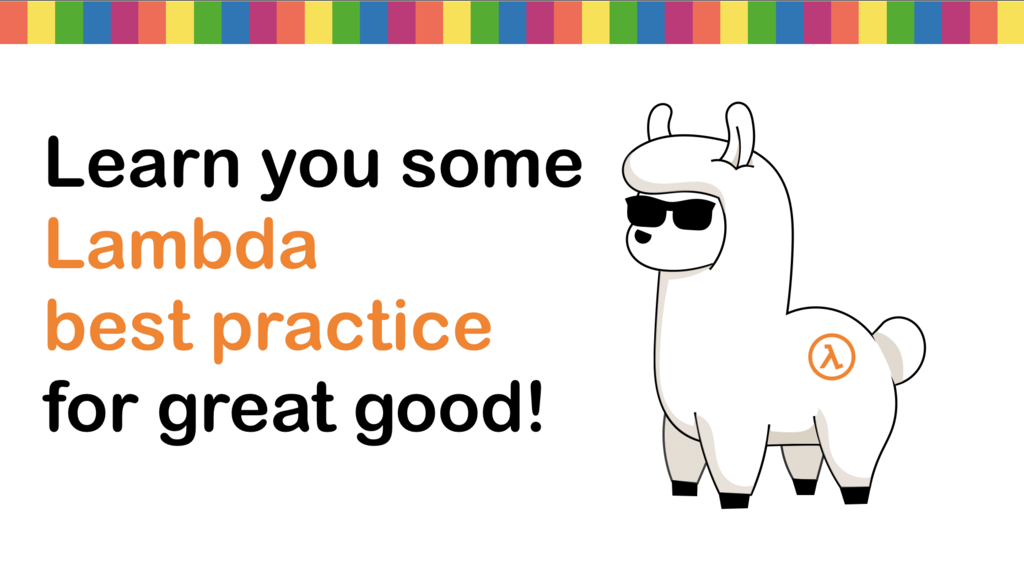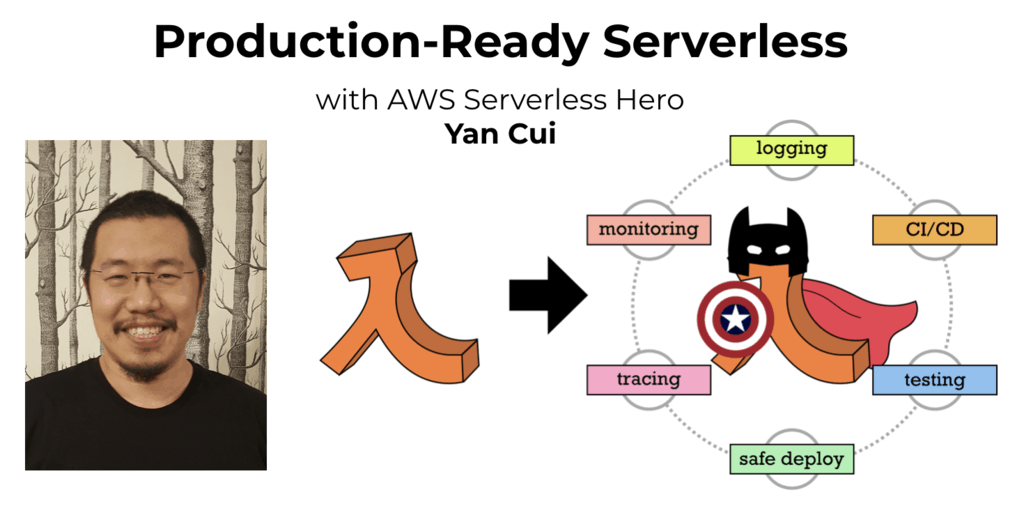# What does this F# code look like in Erlang – Part 1 of N

Check out my new course Learn you some Lambda best practice for great good! and learn the best practices for performance, cost, security, resilience, observability and scalability.

Brushing up on Erlang again having spent so much time with F# since I last looked at Erlang, it’s startling how much parallels I see between the two languages in terms of features and syntax, and I’m sure it’s no coincidenceTo help myself and other F# programmers who’re curious about Erlang get started more easily, I’m going to run a series of blog posts on how F# code would look in Erlang.

Related Posts

Assignment

Assignment in F# is done via the let keyword:

let x = 42

let y = 10.0

let z = x * int y

In Erlang, you just write:

1> X = 42.

42

2> Y = 10.0.

10.0

3> Z = X * Y.

420.0

It’s worth noting that in Erlang, variable names have to start with a capital letter or an underscore (‘_’). Also, Erlang variables can be ‘unbound’, and assigned once:

1> A.

* 1: variable ‘A’ is unbound

2> A = 123.

123

3> A = 321.

** exception error: no match of right hand side value 321

However, you can assign the same value to a bound variable more than once:

1> A = 123.

123

2> A = 123.

123

This works because the equals (‘=’) operator in Erlang is a pattern match operator as opposed to assignment!

The = operator compares values and complains if they’re different, if they are the same it will return the value instead. In the case of an unbound variable, the operator will automatically bind the right-hand side value to the variable on the left-hand side.

Wildcard

In F#, you can use the underscore ‘_’ as a wildcard to ignore the value on the right-hand side of an expression:

let _ = 42

In Erlang, it’s the same thing!

1> _ = 42.

42

Boolean Algebra

F#:

let a = true && true   // true

let b = true || false    // true

let c = not b            // false

Erlang:

1> A = true and true.

true

2> B = true or false.

true

3> C = not B.

false

In F#, the logical and (‘&&’) and logical or (‘||’) operators will only evaluate arguments on both sides of the operator if necessary, so in the following code snippet only f () is evaluated:

let f () = printfn "hello"; true

let g () = printfn "world"; false

let x = f () || g ()      // prints ‘hello’

let y = g () && f ()   // prints ‘world’

In Erlang, the boolean and and or operators will always evaluate arguments on both sides of the operator. If you want short-circuit behaviour, use the andalso and orelse operators instead.

Comparison

F#:

let x = 5 = 5     // true

let y = 5 <> 6   // true

let a = 5 < 6     // true

let b = 5 <= 5   // true

let c = 6 > 5     // true

let d = 5 >= 5   // true

Erlang:

1> 5 =:= 5.

true

2> 5 =/= 6.

true

3> 5 < 6.

true

4> 5 =< 5.

true

5> 6 > 5.

true

6> 5 >= 5.

true

Tuples

In F#, a tuple is written in the form ( Element1, Element2, … ElementN ):

let point = (4, 5)

let x, y = point

In Erlang, a tuple is written in the form { Element1, Element2, …, ElementN }, such as:

1> Point = { 4, 5 }.

{ 4, 5 }

2> { X, Y } = Point.

{ 4, 5 }

Lists

Lists in F# and Erlang are both implemented as linked-lists, whilst F#’s lists can hold elements of the same type, Erlang’s lists can hold a mixture of anything.

F#:

let lst = [ 1; 2; 3; 4 ]      // [ 1; 2; 3; 4 ]

let hd = lst.Head          // hd = 1

let _::tl = lst                 // tl = [ 2; 3; 4 ]

let lst2 = 1::2::3::4::[ ]    // [ 1; 2; 3; 4 ]

Erlang:

1> Lst = [ 1, "one", { 2, 3 } ].

[1,"one",{2,3}]

2> hd(Lst).

1

3> [ _ | Tl ] = Lst.

["one",{2,3}]

4> Tl.

[“one”,{2, 3}]

6> [ 1 | [ 2 | [ 3 | [ 4 | [ ] ] ] ] ].

[1,2,3,4]

List Comprehensions

You can write a simple list comprehension in F# like this:

let lst = [ for x in [ 1..4 ] -> x * 2 ]

This code will look like this in Erlang:

1> Lst = [ X * 2 || X <- [ 1, 2, 3, 4 ] ].

[ 2, 4, 6, 8 ]

Erlang’s list comprehensions also lets you specify conditions on the input for instance:

1> Lst = [ X * 2 || X <- [ 1, 2, 3, 4, 5, 6, 7, 8 ], X =< 4 ].

[ 2, 4, 6, 8 ]

Support for when guards in F#’s list comprehension was removed quite a while back, but you can still achieve the same effect easily enough with a simple if conditions:

let lst = [ for x in [ 1..8 ] do if x <= 4 then yield x * 2 ]

In F#, you can also nest multiple list comprehensions:

let lst = [ for x in [1..8] do

for y in [1..4] do

if x <= 4 then yield x, y]

To do the same in Erlang, you can specify a second ‘generator expression’:

Lst = [ { X, Y } || X <- [ 1, 2, 3, 4, 5, 6, 7, 8 ], Y <- [ 1, 2, 3, 4 ], X =< 4].

In general, Erlang’s list comprehension is of the form: [ Expression || GeneratorExp1, GeneratorExp2, …, GeneratorExpN, Condition1, Condition2, …, ConditionM ].

Functions

In F#, you can define a function using the let keyword followed by the name of the function and its parameters:

let ident x = x

In Erlang, functions can be defined only in modules, and is defined like the following:

-module(my_module).

-export([ident/1]).

ident ( X ) –> X

Lambda (anonymous function)

In F#, you can declare a lambda using the fun keyword:

let add = (fun (x, y) –> x + y);

let ans = add(3, 2);     // ans = 5

Guess what, it’s fun to declare lambdas in Erlang too1> Add = fun (X, Y) –> X + Y end.

#Fun<erl_eval.12.82930912>

5Hi, I’m Yan. I’m an AWS Serverless Hero and the author of Production-Ready Serverless.

I specialise in rapidly transitioning teams to serverless and building production-ready services on AWS.

Are you struggling with serverless or need guidance on best practices? Do you want someone to review your architecture and help you avoid costly mistakes down the line? Whatever the case, I’m here to help.Check out my new course, Learn you some Lambda best practice for great good! In this course, you will learn best practices for working with AWS Lambda in terms of performance, cost, security, scalability, resilience and observability. Enrol now and enjoy a special preorder price of £9.99 (~\$13).Are you working with Serverless and looking for expert training to level-up your skills? Or are you looking for a solid foundation to start from? Look no further, register for my Production-Ready Serverless workshop to learn how to build production-grade Serverless applications!